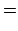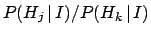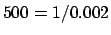Next: Inferring numerical values of Up: Bayesian inference for simple Previous: Bayes' theorem

## Inference for simple hypotheses

Making use of formulae (20) or (21), we can easily solve many classical problems involving inference when many hypotheses can produce the same single effect. Consider the case of interpreting the results of a test for the HIV virus applied to a randomly chosen European. Clinical tests are very seldom perfect. Suppose that the test accurately detects infection, but has a false-positive rate of 0.2%:If the test is positive, can we conclude that the particular person is infected with a probability of 99.8% because the test has only a 0.2% chance of mistake? Certainly not! This kind of mistake is often made by those who are not used to Bayesian reasoning, including scientists who make inferences in their own field of expertise. The correct answer depends on what we else know about the person tested, that is, the background information. Thus, we have to consider the incidence of the HIV virus in Europe, and possibly, information about the lifestyle of the individual. For details, see (D'Agostini 1999c).

To better understand the updating mechanism, let us take the ratio of Eq. (20) for two hypothesesand(23)

where the sums in the denominators of Eq. (20) cancel. It is convenient to interpret the ratio of probabilities, given the same condition, as betting odds. This is best done formally in the de Finetti approach, but the basic idea is what everyone is used to: the amount of money that one is willing to bet on an event is proportional to the degree to which one expects that event will happen. Equation (23) tells us that, when new information is available, the initial odds are updated by the ratio of the likelihoods, which is known as the Bayes factor.

In the case of the HIV test, the initial odds for an arbitrarily chosen European to be infectedare so small that we need a very high Bayes' factor to be reasonably certain that, when the test is positive, the person is really infected. With the numbers used in this example, the Bayes factor is. For example, if we take for the prior, the Bayes' factor changes these odds to, or equivalently, the probability that the person is infected would be, quite different from theanswer usually prompted by those who have a standard statistical education. This example can be translated straightforwardly to physical problems, like particle identification in the analysis of a Cherenkov detector data, as done, e.g. in (D'Agostini 1999c).Next: Inferring numerical values of Up: Bayesian inference for simple Previous: Bayes' theorem
Giulio D'Agostini 2003-05-13